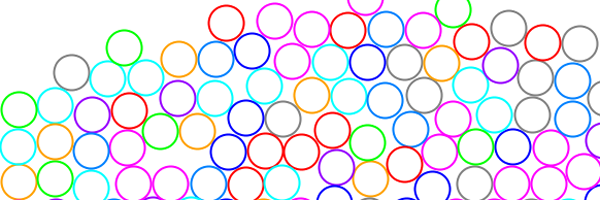Circle-Circle Collision Tutorial

Source Code

Submitted to the 2010 Intel Science Talent Search.

Presented at the 2010 Junior Science and Humanities Symposium

Introduction

Circle-circle collision modeling is an important component of many simulations and games today. For some software, accuracy takes precedence over speed, and thus perfect circles, rather than polygons or bounding boxes, must be used, in addition to continuous collision detection. This means that there should be no allowance for circles going "through" other circles, a guarantee paid with extra computational time. Instances where this extra accuracy may be useful include pool, air hockey, and golf. Fortunately, between circles, such extra computation is minor, as collision detection and response between moving circles is mostly linear algebra and algebraic manipulation.

The algorithm in this tutorial is intended to accurately find the location of the collision and calculate the resultant velocity without using discrete time steps (moving the circle forward until a collision occurs). Thus the algorithm works with dynamic circles that respond to impulse forces. A continuous a priori approach to collision detection is used, meaning a collision is found before it occurs and then corrected. A posteriori approaches are discrete, using only the static circle collision portion of this algorithm, and only detect a collision after it occurs. Such an approach may be faster, but is inherently inaccurate.

This tutorial assumes basic knowledge of a programming language (Java in particular) and knowledge of basic algebra and geometry. Circles in this algorithm are assumed to be non-rotating.

Object Definitions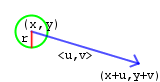Moving circle (circle) Initial position (x, y) Velocity described by its componentsRadius r Mass m The movement vector is .

The Closest Point on a Line to a Point Algorithm

The closest point on a line to a point algorithm does exactly what its name says - it finds the closest point on the given line segment to the given point. It is an extension of the line-line intersection algorithm and is used to find when a collision occurs and where it occurs, and is quite critical to the circle-circle collision detection algorithm.

Steps

1. Start with a given point (x0, y0) and a given line segment, described by endpoints (lx1, ly1) and (lx2, ly2).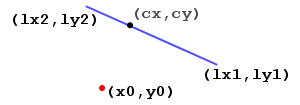2. Take the endpoints of the line segment and turn it into an equation of the form Ax + By = C.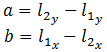3. The equation of the line perpendicular to the initial line segment is given by  -Bx + Ay = C, but this time (x, y) is the given point so that the new equation crosses through the given point.4. Find the determinant of the two equations algebraically: determinant = A*A + B*B.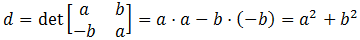5. Use Cramer's Rule to solve for the point of intersection of the original line and the perpendicular line, and that gives us the closest point on the given line to given point.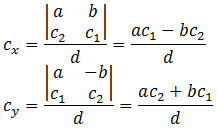If the determinant = 0 , then the point is on the line, and thus the closest point on the line to the point is the point itself!

Java code

Point closestpointonline(float lx1, float ly1,
float lx2, float ly2, float x0, float y0){
float A1 = ly2 - ly1;
float B1 = lx1 - lx2;
double C1 = (ly2 - ly1)*lx1 + (lx1 - lx2)*ly1;
double C2 = -B1*x0 + A1*y0;
double det = A1*A1 - -B1*B1;
double cx = 0;
double cy = 0;
if(det != 0){
cx = (float)((A1*C1 - B1*C2)/det);
cy = (float)((A1*C2 - -B1*C1)/det);
}else{
cx = x0;
cy = y0;
}
return new Point(cx, cy);
}

Static Circle Collision

Before moving circles are considered, the simpler case of two non-moving circles should be considered first. A collision in this case is actually an intersection of the radii, or an overlap between the areas of the two circles.

Even though when circles are moving and the moving circle collision algorithm works correctly, then an overlap should never occur, but calculations are not always precise and the occasional overlap may occur. Whether or not this comes into play depends on the method used to select objects for collision testing.

Static Circle-Circle Collision Detection

Determining whether or not two circles intersect or overlap is the most basic way of determining whether or not two circles have collided. This is done by comparing the distance squared between the two circles to the radius squared between the two circles.

Steps

1. Find the distance between the centers of the two circles using the distance formula: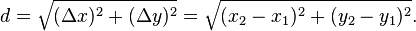Distance is also the magnitude of a vector, denoted by the double vertical bars on both sides of the vector, as seen below. This fact will be used later to simplify mathematical expressions (so that the distance formula isn't written over and over again).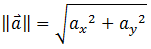2. Then the distance squared is compared with the radii squared.
• If distance^2 = radius^2, then the circles are just touching each other, and thus not intersecting or overlapping.
• If distance^2 < radius^2, then the circles are intersecting.
• If distance^2 > radius^2, then the circles are not intersecting.

Java code

boolean checkcirclecollide(double x1, double y1, float r1, double x2, double y2, float r2){
return Math.abs((x1 - x2) * (x1 - x2) + (y1 - y2) * (y1 - y2)) < (r1 + r2) * (r1 + r2);
}

Static Circle-Circle Collision Response

Once an intersection is found, the circles must be moved away from each other so that they no longer overlap.

StepsFind the midpoint between the two circles. This is done by averaging the centers of the two points (source). If the midpoint is p and the centers of the circles are denoted by c1 and c2, then:Java code

midpointx = (circle1.x + circle2.x) / 2;
midpointy = (circle1.y + circle2.y) / 2;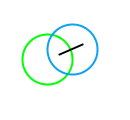Set the new centers of the circles to be the radius (R) away from p along the line that connects the centers of the two radii. So if cf denotes the final position of the circle, then for each circle: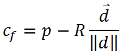The vector d denotes the vector from the radius of one circle to the other. The negative sign is there because we want to move away from the midpoint so that the circles no longer intersect. The magnitude of d is the distance from one circle to the other. The vector over its magnitude "normalizes" the vector.

Java code

circle1.x = midpointx + circle1.radius * (circle1.x - circle2.x) / dist;
circle1.y = midpointy + circle1.radius * (circle1.y - circle2.y) / dist;
circle2.x = midpointx + circle2.radius * (circle2.x - circle1.x) / dist;
circle2.y = midpointy + circle2.radius * (circle2.y - circle1.y) / dist;

Dynamic Circle - Static Circle Collision

When one circle is moving, the situation becomes more complex and determining the location of the collision is no longer trivial. Linear algebra can be used to compare the velocity of the moving circle with the position of the non-moving circle. Thus whether or not a collision occurs can be determined and even the exact location. Then, with the use of physics and more algebra, the resultant velocity can be found.

Dynamic-Static Circle Collision Detection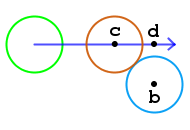One way to think of collision detection between a dynamic circle and a static circle is checking whether or not the area swept out by the moving circle overlaps the static circle. Unfortunately, this is difficult to calculate and doesn't give the location of the collision. Another way is to use linear algebra to find the location of the collision, and if that isn't possible, then no collision has occurred.

Steps

1. Find the closest point on the movement vector of the moving circle (green) to the center of the non-moving circle (b). This point is d on the diagram. Then find the distance between the closest point and the center of the non-moving circle (b to d).

• If it is larger than or equal to the sum of the radii, then no collision has occurred.

• If it is smaller than the sum of the sum of the radii, then a collision has occurred.

Note that only the squares of the distances need to be compared, so a square root is not necessary.

Java code

Point d = closestpointonline(circle1.x, circle1.y,
circle1.x + circle1.vx, circle1.y + circle1.vy, circle2.x, circle2.y);
double closestdistsq = Math.pow(circle2.x - d.x, 2) + Math.pow(circle2.y - d.y), 2);
// a collision has occurred
}else{
// no collision has occurred
}
2. If a collision has occurred, then the Pythagorean theorem gives us the point of collision, c.Manipulating it gives us one of the sides: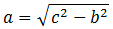Then plugging in gives us (note that b - c is the sum of the radii):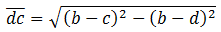Subtracting d by the above value multiplied by the norm of the movement vector ( v ) then gives us c :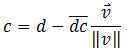Java code

double backdist = Math.sqrt(Math.pow(circle1.radius + circle2.radius, 2) - closestdistsq);
double movementvectorlength = Math.sqrt(Math.pow(circle1.vx, 2) + Math.pow(circle1.vy, 2));
double c_x = d.x - backdist * (circle1.vx / movementvectorlength);
double c_y = d.y - backdist * (circle1.vy / movementvectorlength);

Dynamic-Static Circle Collision ResponseCollision response consists of solving momentum and kinetic energy equations for the final velocity of the object. All collision conserve momentum, while elastic collisions conserve kinetic energy in addition to momentum. The law of conservation of momentum states that the total momentum, or mass times velocity, of a system before a collision is equal to the total momentum after the collision. This is stated in the equation below: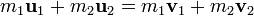Conservation of energy, is a bit more complicated. Energy is half of mass times velocity squared, and follows the same principle, it is the same before and after a collision if the collision is elastic. Elastic collisions lose no energy.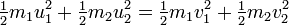Steps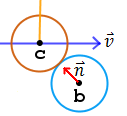1. Find the norm of the vector from the point of collision (c) to the center of the second circle (b).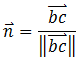2. Find a value, p, that relates the velocity of the first circle (v), with the masses of the two objectsThe dot product of two vectors is: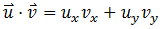3. Then combine the two values to find the resultant velocity of the first circle, w.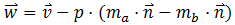Java code

double collisiondist = Math.sqrt(Math.pow(circle2.x - c_x, 2) + Math.pow(circle2.y - c_y, 2);
double n_x = (circle2.x - c_x) / collisiondist;
double n_y = (circle2.y - c_y) / collisiondist;
double p = 2 * (circle1.vx * n_x + circle1.vy * n_y) /
(circle1.mass + circle2.mass); double w_x = circle1.vx - p * circle1.mass * n_x - p * circle2.mass * n_x; double w_y = circle2.vy - p * circle1.mass * n_y - p * circle2.mass * n_y;

Dynamic Circle-Circle Collision

Going from one moving circle to two moving circles is easy - just change the frame of reference to one circle, since the circles are moving at constant velocity. Do the collision detection and response, then switch everything back to the original frame of reference. What does change is the equation for collision response, because the velocity of the second circle must be taken into account.

Note that the notation changes from a for the first circle to 1. The second circle is 2.

Steps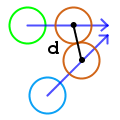1. Find the distance between the two points of collision.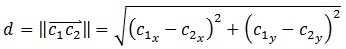2. Find the norm of the vector from the point of collision for the first circle and the point of collision of the second circle.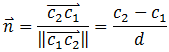3. Calculate the p-value that takes into account the velocities of both circles.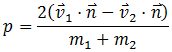4. Calculate the final velocity of each circle using each p-value. Note that each resultant is just the opposite sign with each variable replaced with the corresponding variable.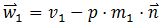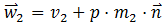Java code

double d = Math.sqrt(Math.pow(cx1 - cx2, 2) + Math.pow(cy1 - cy2, 2));
double nx = (cx2 - cx1) / d;
double ny = (cy2 - cy1) / d;
double p = 2 * (circle1.vx * nx + circle1.vy * n_y - circle2.vx * nx - circle2.vy * n_y) /
(circle1.mass + circle2.mass);
vx1 = circle1.vx - p * circle1.mass * n_x;
vy1 = circle1.vy - p * circle1.mass * n_y;
vx2 = circle2.vx + p * circle2.mass * n_x;
vy2 = circle2.vy + p * circle2.mass * n_y;

Conclusion

Starting from the simplest case of two non-moving (static) circles, and moving up to two moving circles, we have developed an algorithm for circle-circle collision detection and response. Most of the time, the above algorithms are combined into one to cover nearly all possible cases. Such a task consists of checking the velocity of each circle and using the appropriate algorithm for the situation. Once you've done that, you've got a very accurate circle-circle collision simulator! To fully utilize the algorithm, it should be in some sort of loop that selects the circles to be compared. Often this is simply just a doubly-nested for loop that goes through the list of circles, running the collision detection algorithm on each of them.

Precision and accuracy were the highest priority in the development of this algorithm. If speed is a concern, bounding boxes may be preferable to bounding circles, especially if sprites are used. Otherwise, pruning methods used to reduce the number of collision tests necessary would certainly reduce the impact of this algorithm in a time-critical application.

One may note that this algorithm only works for two circles that are colliding. What happens when there is more than two circles in a simulation? This is the focus of my paper, which treats each collision as independent and considers the case of one circle colliding into multiple circles. Yet it doesn't cover another issue: what happens when more than two circles collide at the same time? That, as of now, is an unsolved problem.